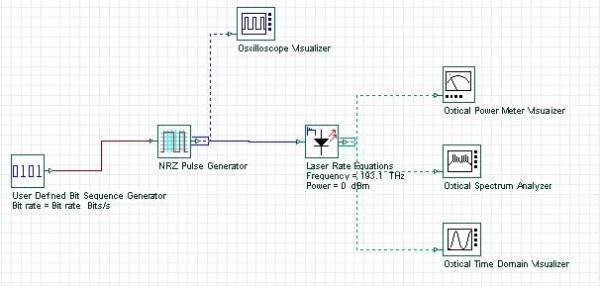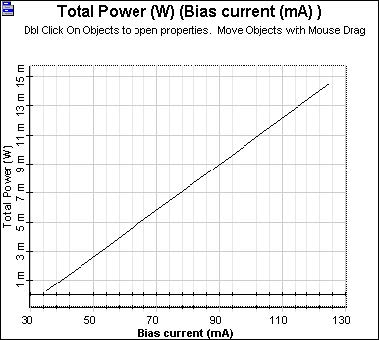The Light – Current (L-I) curve characterizes the emission properties of a semiconductor laser as it shows the current that needs to be applied to obtain a certain amount of power.

We will show a L-I curve of our laser rate equation model with default Ith = 33.45mA . The project is depicted in Figure 1.Figure 1: Project layout

(In fact the bit sequence generator and NRZ generator could be disabled, as the results are the same.)

Discussion of the numerical parameters: bit rate is 2.5Gb/s and sequence length 8 bits, therefore, the time window is about 3.2ns. Samples per bit are 64, therefore, the sample rate is 160GHz. The default resolution therefore is 312MHz.

The L-I curve is generated after sweeping the parameter bias current from 35 to 125 mA. Modulation peak current = 0.Figure 2: L-I curve—Power vs. bias

Linear dependence on emitted power on the bias current can be well seen.